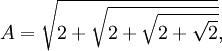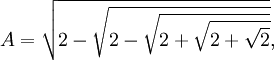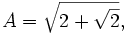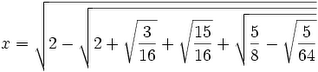## Sunday, January 21, 2007

### Solving Van Roomen's Problem: Step Five

In today's blog, I show how we can use the trigonometric identities in my previous blog to simplify Van Roomen's x and A examples. To see the statement of Van Roomen's problem, start here.

The content in today's blog is taken from Jean-Pierre Tignol's Galois' Theory of Algebraic Equations.

Lemma 1:= 2 sin(15π/25)

Proof:

(1) cos(90°) = 0 [See Property 7, here]

(2) cos(2π/4) = cos(π/2) = cos(90°) = 0 [See here for review of radians]

(3) 2cos(π/4) = ±√2 + 2cos(π/2) [See Lemma 1, here]

(4) So, 2cos(π/4) = ±√2

(5) 2cos(π/8) = ±√2 + 2cos(π/4) = ±√2 + √2

(6) 2cos(π/16) = ±√2 + 2cos(π/8) = ±√2 + √(2 + √2)

(7) 2cos(π/32) = ±√2 + 2cos(π/16) = ±√2 + √[2 + √(2 + √2)]

(8) 2cos(π/32) = 2sin(π/2 - π/32) [See Lemma 1, here]

(9) 2sin(π/2 - π/32) = 2sin(16π/32 - π/32) = 2sin(15π/32) = 2sin(15π/25)

QED

Lemma 2:= 2 sin(π/[25*3])

Proof:

(1) 2cos(π/6) = √3 [See Corollary 1.1, here]

(2) 2cos(π/12) = ±√2 + 2cos(π/6) [See Lemma 1, here]

(3) 2cos(π/12) = ±√2 + √3

(4) 2cos(π/24) = ±√2 + 2cos(π/12) = ±√2 + √(2 + √3)

(5) 2cos(π/48) = ±√2 + 2cos(π/24) = ±√2 + √[2 + √(2 + √3)]

(5) 2sin(π/96) = ±√2 - 2cos(π/48) [See Lemma 2, here]

(6) 2sin(π/96) = 2sin(π/[25*3]) = ±√2 - {2 + √[2 + √(2 + √3)]}

QED

Lemma 3:= 2 sin(15π/26)

Proof:

(1) From step#6 in Lemma 1 above, we have:

2cos(π/16) = ±√2 + √(2 + √2)

(2) Using Lemma 2 here, we have:

2sin(π/32) = ±√2 - 2cos(π/16) = ±√2 - √[2 + √(2 + √2)]

(3) 2cos(π/2 - π/32) = 2sin(π/32) [See Corollary 1.1, here]

(4) 2cos(π/2 - π/32) = 2cos(16π/32 - π/32) = 2cos(15π/32)

(5) 2sin(15π/64) = ±√2 - 2cos(π/16) = ±√2 - √{2 - √[2 + √(2 + √2)]}

(6) 2sin(15π/64) = 2sin(15π/)

QED

Lemma 4:= 2sin(π/[26*3])

Proof:

(1) Using step #5 from Lemma 2 above, we have:

2cos(π/24*3) = ±√2 + 2cos(π/24) = ±√2 + √[2 + √(2 + √3)]

(2) So we have:

2cos(π/25*3) = ±√2 + 2cos(π/24*3) = ±2 + {2 + √[2 + √(2 + √3)]}

(3) And also:

2sin(π/26*3) = ±√2 - 2cos(π/25*3) =

= ±
2 - √(2 + {2 + √[2 + √(2 + √3)]})

QED

Lemma 5:= 2sin(3π/23)

Proof:

(1) From Lemma 1 above, step #5, we have:

2cos(π/8) = ±√2 + 2cos(π/4) = ±√2 + √2

(3) Using Lemma 1 here we have:

2cos(π/8) = 2sin(π/2 - π/8) = 2sin(4π/8 - π/8) = 2sin(3π/8) = 2sin(3π/23)

QED

Lemma 6:= 2sin(π/[23*3*5])

Proof:

(1) π/5 - π/6 = 6π/30 - 5π/30 = π/30

(2) 2cos(π/30) = 2cos(π/5 - π/6) = 2cos(π/5)cos(π/6) + 2sin(π/5)sin(π/6) [See Theorem 2, here for cosine addition/subtraction rule]

(3) Using previous results, we can compute cos(π/10) and sin(π/10):

cos(π/10) = sin(π/2 - π/10) = sin(5π/10 - π/10) = sin(4π10) = sin(2π/5) [See here Lemma 1,here]

sin(2π/5) = (1/2)(5 + √5)/2 [See Lemma 6, here]

sin(π/10) = cos(π/2 - π/10) = cos(2π/10) [See Corollary 1.1, here]

cos(2π/5) = (√5 - 1)/4 [See Lemma 5, here]

(4) Now, we can use cos(π/10), sin(π/10) to compute cos(π/5) and sin(π/5):

Using the cosine double angle formula (see Corollary 3.1, here):
cos(2x) = 2cos2(x) - 1

So,
cos(2*π/10) = cos(π/5) = 2cos2(π/10) - 1 = (2)(1/8)[5 + √5] - 1 = 5/4 + (√5)/4 - 4/4 = (√5 + 1)/4

Now, we can use sin2(π/5) + cos2(π/5) = 1 [see Corollary 2, here], to get:

sin(π/5)2 = 1 - cos2(π/5) = 16/16 - (1/16)(5 + 2√5 + 1) = (1/16)[10 - 2√5 ]

This gives us:
sin(π/5) = (1/4)√10 - 2√5

(5) Using a previous result (see Corollary 1.2, here), we know that:

cos(π/6) = cos(30°) = √3/2

sin(π/6) = sin(30 °) = (1/2)

(6) So, this gives us that:

cos(π/30) = 2[(√5 + 1)/4][3/2] + 2[(1/4)√10 - 2√5][(1/2)] =

= (1/4)[15 + 3] + (1/4)[10 - 2√5] =(3/16) + (15/16) + (5/8) - √(5/64).

(7) 2cos(π/[22*5*3]) = ±√2 + 2cos(π/30) [See Lemma 1, here]

So that:
2cos(π/[22*5*3]) = ±√{2 + (3/16) + (15/16) + [(5/8) - √(5/64)]}

(8) 2sin(π/[23*5*3]) = ±√2 - 2cos(π/[22*5*3]) [See Lemma 2, here]

So:
2sin(π/[23*5*3]) = ±√2 - √{2 + (3/16) + (15/16) + [(5/8) - √(5/64)]}

QED

Lemma 7:= 2 sin(π/[3*5])

Proof:

(1) 2sin(π/15) = (1/2)√7 - √5 - √(30 - 6√5) [See Corollary 7.1, here]

(2) Since (1/2) = (1/√4), we have:

2sin(π/15) = √(7/4) - √(5/16) - √[(30 - √[36*5])/16] =

= √(7/4) - √(5/16) - √[15/8 - √(36*5/16*16)] =

= √(7/4) - √(5/16) - √[15/8 - √(9*5/4*16)] =

= √(7/4) - √(5/16) - √[15/8 - √(45/64)]

QED

References
• Jean-Pierre Tignol, Galois' Theory of Algebraic Equations, World Scientific, 2001